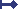Zur Übersicht - INFO - Neueste 50 Beiträge - Neuer Beitrag - Suchen - Zum C-Control-I-Forum - Zum C-Control-II-Forum

Timer_T1Time and Timer_T3Time do not work as they should Kategorie: IDE (von PepeV - 19.02.2010 17:46)
 Ich nutze: C-Control Pro Mega128, Pro-Bot128
Hi all (and especially Peter),

The functions Timer_T1Time(word Time,byte PS) and Timer_T3Time(word Time,byte PS) generate
interrupts with a frequency that does not match the parameters PS and Time. It seems that the value
of Time is totally irrelevant and that the value of PS sets the prescaler values of the Mega32 while
I compile for Mega128.
In contrast, the function Timer_T0Time(byte Time,byte PS) does what should do for all values of PS and
Time.
Below is the program I used to test it and the results.

Is this an error in the Mega128 or what?

Regards,
Pepe

word T0_Cnt, T1_Cnt, T2_Cnt, T3_Cnt;

void main(void)
{
Irq_SetVect(INT_TIM0COMP,T0_ISR);
Irq_SetVect(INT_TIM1CMPA,T1_ISR);
Irq_SetVect(INT_TIM2COMP,T2_ISR);
Irq_SetVect(INT_TIM3CMPA,T3_ISR);

Timer_T0Time(144,PS0_256);   // 400 Hz
Timer_T1Time(12345,PS0_32);  // Only the prescaler value matters. Why?!
// Timer T2 freq is allways 100 Hz
Timer_T3Time(54321,PS0_64);  // Only the prescaler value matters. Why?!

while (T2_Cnt<10000);   // run 100 sec

Msg_WriteFloat(T0_Cnt/100.0);
Msg_WriteChar(13);
Msg_WriteFloat(T1_Cnt/100.0);
Msg_WriteChar(13);
Msg_WriteFloat(T2_Cnt/100.0);
Msg_WriteChar(13);
Msg_WriteFloat(T3_Cnt/100.0);
Msg_WriteChar(13);
}

void T0_ISR(void)
{
int irqcnt;
T0_Cnt++;
irqcnt=Irq_GetCount(INT_TIM0COMP);
}

void T1_ISR(void)
{
int irqcnt;
T1_Cnt++;
irqcnt=Irq_GetCount(INT_TIM1CMPA);
}

void T2_ISR(void)
{
int irqcnt;
T2_Cnt++;
irqcnt=Irq_GetCount(INT_TIM2COMP);
}

void T3_ISR(void)
{
int irqcnt;
T3_Cnt++;
irqcnt=Irq_GetCount(INT_TIM3CMPA);
}

The results:
Timer_T1Time(xxxxx,PS0_1)      f=225,01 Hz   (matches Timer=65535 and PS=1)
Timer_T1Time(xxxxx,PS0_8)      f= 28,12 Hz    (matches Timer=65535 and PS=8)
Timer_T1Time(xxxxx,PS0_32)    f=  3,51 Hz     (matches Timer=65535 and PS=64)
Timer_T1Time(xxxxx,PS0_64)    f=  0,88 Hz     (matches Timer=65535 and PS=256)
Timer_T1Time(xxxxx,PS0_128)   f=  0,21 Hz    (matches Timer=65535 and PS=1024)

Antwort schreiben

Antworten:Re: Timer_T1Time and Timer_T3Time do not work as they should (von PeterS - 20.04.2010 17:46)Re: Timer_T1Time and Timer_T3Time do not work as they should (von Conny - 10.04.2010 15:52)